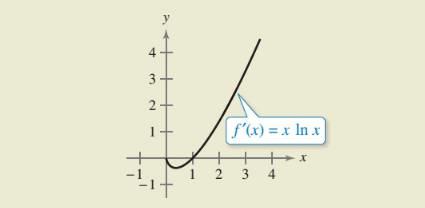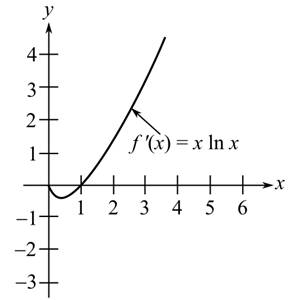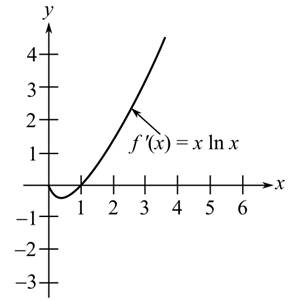Chapter 8.2, Problem 62E

Chapter
Section
Textbook Problem

# HOW DO YOU SEE IT? Use the graph of f' shown in the figure to answer the following.(a) Approximate the slope of f at x = 2 . Explain.(b) Approximate any open intervals on which the graph of f is increasing and any open intervals on which it is decreasing. Explain.

(a)

To determine

To calculate: The slope for the function f at the point x=2 where f' is represented by the graph as follows:Explanation

Given:

The graph of f:

Formula used:

The slope of a function h(x) at the point (x1,y1) is (ddxh(x))(x1,y1)

Calculation:

It is known that the derivative of a function is equivalent to its slope

(b)

To determine

To calculate: The open intervals in where the graph for the function f is increasing and decreasing, Where f' is represented by the graph as follows:### Still sussing out bartleby?

Check out a sample textbook solution.

See a sample solution

#### The Solution to Your Study Problems

Bartleby provides explanations to thousands of textbook problems written by our experts, many with advanced degrees!

Get Started

#### Use Exercise 92 to evaluate the integral 0xsinx1+cos2xdx

Single Variable Calculus: Early Transcendentals, Volume I

#### In problems 37-44, perform the indicated operations and simplify. 37.

Mathematical Applications for the Management, Life, and Social Sciences

#### Find y if x6 + y6 = 1.

Single Variable Calculus: Early Transcendentals